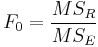# ANOVA Calculations in Multiple Linear Regression

 Appendix A ANOVA Calculations in Multiple Linear Regression

## Contents

The sum of squares for the analysis of variance in multiple linear regression is obtained using the same relations as those in simple linear regression, except that the matrix notation is preferred in the case of multiple linear regression. In the case of both the simple and multiple linear regression models, once the observed and fitted values are available, the sum of squares are calculated in an identical manner. The difference between the two models lies in the way the fitted values are obtained. In a simple linear regression model, the fitted values are obtained from a model having only one predictor variable. In multiple linear regression analysis, the model used to obtained the fitted values contains more than one predictor variable.

## Total Sum of Squares

Recall from Simple Linear Regression Analysis that the total sum of squares,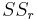, is obtained using the following equation: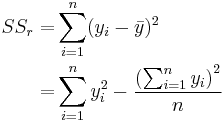The first term,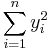, can be expressed in matrix notation using the vector of observed values, y, as: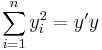If J represents an n x n square matrix of ones, then the second term,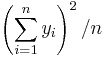, can be expressed in matrix notation as: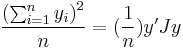Therefore, the total sum of squares in matrix notation is: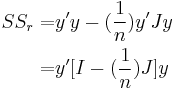where I is the identity matrix of order.

## Model Sum of Squares

Similarly, the model sum of squares or the regression sum of squares,, can be obtained in matrix notation as: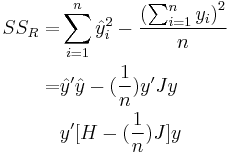where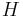is the hat matrix and is calculated using.

## Error Sum of Squares

The error sum of squares or the residual sum of squares,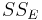, is obtained in the matrix notation from the vector of residuals,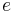, as:## Mean Squares

Mean squares are obtained by dividing the sum of squares with their associated degrees of freedom. The number of degrees of freedom associated with the total sum of squares,, is (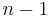) since there are n observations in all, but one degree of freedom is lost in the calculation of the sample mean,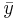. The total mean square is: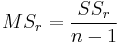The number of degrees of freedom associated with the regression sum of squares,, is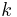. There are (k+1) degrees of freedom associated with a regression model with (k+1) coefficients,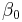,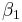,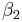....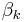. However, one degree of freedom is lost because the deviations, (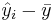), are subjected to the constraints that they must sum to zero (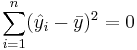). The regression mean square is:The number of degrees of freedom associated with the error sum of squares is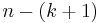, as there areobservations in all, but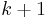degrees of freedom are lost in obtaining the estimates of,,...to calculate the predicted values,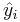. The error mean square is: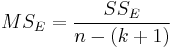The error mean square,, is an estimate of the variance,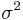, of the random error terms,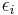.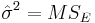## Calculation of the Statistic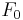Once the mean squares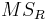andare known, the statistic to test the significance of regression can be calculated as follows: